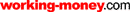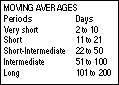HOT TOPICS LIST

LIST OF TOPICS

# Why So Many Moving Averages?

03/28/07 01:28:21 PM PST
by Rudy Teseo

So many to choose from...so little time.

 If you're a student of technical analysis, it is highly probable that the first indicator you learned to use was the simple moving average (MA). Back before technical analysis computer programs, I used to manually plot a simple moving average of my stock on graph paper. This may sound stone-age primitive now, but it was effective then. Through the years, in my studies of technical analysis, I have found references to no less than seven types of moving averages in the literature. (If you know of more, please email me the details, thank you.) These days, moving averages are the most common indicators used in technical analysis and form the basis of other indicators. For example, the famous moving average convergence/divergence (MACD) is a combination of several MAs. THE MANY MOVING AVERAGES When I started studying technical analysis, I was intrigued with the different types of moving averages. Why are there so many? As I learned more about MAs the answer became simple enough: different strokes for different folks. Over the years, technicians and programmers have attempted to improve the MA so it can do a better job of what it's supposed to do. Moving averages smooth data and provide a clearer picture of the trends and reversals of the price of a security. Moving averages together with price, or two moving averages together, are useful for identifying entry and exit points. However, these signals tend to lag as they are based upon past performance. So techniques were developed to produce signals with fewer lags and so with more accuracy.What makes these moving averages different is the weight applied to the more recent data. The seven types of MAs are:Simple moving average = SMA Weighted moving average = WMA Exponential moving average = EMA Triangle moving average = TMA Time series moving average = TSMA Variable moving average = VMA Volume-adjusted moving average = VAMA Simple moving average: The SMA treats all data equally and is simply an average of prices for a selected period (days, weeks, months), which changes with each new period. As today's price is added, the oldest day's price is dropped. One of the uses of the SMA, which has the most lag among the moving averages, is as a buy/sell indicator. When the price moves above the MA you buy; when the price moves below the MA you sell. The inherent lag causes you to get in late and out late. Using shorter time periods reduces the lag but leads to more whipsaws. As with all averages, the time period selected has a great deal to do with the results you attain. The time period you select should fit the (stock) market cycle you are following. Every stock, commodity, and index has a predominant cycle — the rhythmic movement of price swings between highs and lows. Cycles occur in some derivative of the numbers 3 and 4. The predominant cycles are three- to four-week cycles, three- to four-month cycles, six- to eight-month cycles, and three- to four-year cycles. Further, cycle durations are fixed to their corresponding vehicle. If an index has a four-month cycle, it will never be three-month cycle or a six-month cycle. If a stock has a three-month cycle, it will never be a four-month or an eight-month. The ideal MA can be calculated as:MA = (cycle length/2) +1Thus, for a 30-period cycle you would use a moving average of 16. Of course, you have to make sure that moving averages are right for the market you are following. In Technical Analysis Explained, Martin Pring states that moving averages are virtually useless in a trading (congestion) range market since they move right through the middle of the price fluctuations and almost always result in unprofitable signals.Weighted moving average: The WMA applies more weight to later data through mathematical computations (multiplying previous data by a weighting factor based upon the number of periods in the average). The reasoning is that the latest data has more importance than earlier data. Fortunately, most charting programs have these moving averages built in so you don't have to do the math. Like the SMA, the oldest data is dropped when the latest data is added. Exponential moving average: The EMA is more complicated. Like the WMA it gives greater weight to the latest data, but unlike the former two moving averages, it does not drop the oldest data. The type of moving average used in the MACD and the McClellan oscillator, the EMA considers all the data in its history. Calculations for this average are even more complex than the WMA. It is calculated by applying a percentage of today's close to yesterday's average. These percentages can be changed (if you own a program that gives you the flexibility to do it), but in most programs the percentage is fixed and the only parameter you can change is the period. If your program allows you to build custom indicators, the following formula will provide the smoothing constant:SC = 2/(period + 1) Where SC is expressed as a percentageThus, for a 20-day EMA the latest price is weighted at 9.5% of the total instead of the 5% that it would be in calculating the SMA. If your program requires the input in percentages, this formula will convert any period you want to use into percentage. If you need to convert percentage to period, use the following formula:Period = (2/percentage) - 1The use of this formula can be seen in the MACD. Gerald Appel's original MACD specified 15% and 7.5% EMAs. The industry soon changed this to 12-and 26-day MA, respectively, which are today's default values in almost all charting programs.Triangle moving average: The TMA gives more weight to the middle data. This moving average is actually an SMA of an SMA. Time series moving average: The TSMA is similar to the time series forecast. This indicator displays the statistical trend of a stock's price. This MA does not have as much delay in following price changes as the indicator is fitting itself to the data. Variable moving average: The VMA weighs data according to the volatility: as volatility increases, more weight is given to recent data. This is accomplished by adjusting the smoothing constant (percentage) of an EMA. The more volatile the data, the more weight given to the current data. This automatic adjustment allows the average to perform better in both trading and trending markets. In trading markets there are many whipsaws with an SMA, but fewer with the VMA. In trending markets there is quite a lag with an SMA, but much less with the VMA.Volume-adjusted moving average: The VAMA incorporates a volume weighting into the calculations. The days with the higher volume get the greater weight. WHICH MA DO YOU USE? This information is all very interesting, but does it help the technician choose the best type of average? Not in itself. Like most things in technical analysis, where there are options, you have to resort to experimentation and backtesting before you gather enough data to make a decision. One of the popular uses of the MA is the moving average crossover, wherein two MAs are used to identify buy and sell signals. When the fast MA crosses above the slow MA you buy, and when the fast MA crosses below the slow MA you sell. The selection of the periods is your call, based upon the time frame you wish to trade. The table you see in Figure 1 is one I have compiled by averaging many recommendations from texts and articles by recognized authors. It is merely a suggestion, so don't change anything that's working for you.FIGURE 1: MOVING AVERAGE PERIODSNow select a pair from the table that fits your immediate time frame and test it until you have determined that it will produce the trading signals you would like. Try different pairs until you're satisfied you have the best.The typical MA crossover uses one type of moving average with different time periods. But suppose we try two or three different MAs with the same time period as shown in Figure 2. In Figure 2, see the daily chart of Boeing with the three most popular MAs set to 100 days. You get an immediate picture of the different crossovers of the price and the various MAs. An uptrend crosses the EMA before the WMA, and a downtrend crosses the WMA before the EMA. Finding the right combination might allow you to get in a few days (or dollars) sooner, and get out a few days (dollars) sooner. Be aware that the spacing between the averages changes with the stock selected, and that the averages may switch positions.FIGURE 2: BOEING CHART SHOWING THREE DIFFERENT TYPE MOVING AVERAGESFinally, I'd like to mention two more moving averages; the double EMA (DEMA) and the triple EMA (TEMA). These were developed by Patrick Mulloy and discussed in his articles in the January and February 1994 issues of Technical Analysis of STOCKS & COMMODITIES. The author tested DEMA- and TEMA-modified MACD, and achieved much better results and less lag. SUGGESTED READING Appel, Gerald . The Moving Average Convergence-Divergence Trading Method, Advanced Version, Scientific Investment Systems. Mulloy, Patrick G. . "Smoothing Data With Faster Moving Averages," Technical Analysis of STOCKS & COMMODITIES, Volume 12: January. _____ . "Smoothing Data With Less Lag," Technical Analysis of STOCKS & COMMODITIES, Volume 12: February. Pring, Martin J. . Technical Analysis Explained, McGraw-Hill Book Co.

Rudy Teseo

Rudy Teseo is a private investor who trades stocks, options, and currencies. He has taught classes in technical analysis and option trading. He may be reached at rftess@optonline.net.

 Comments or Questions? Article Usefulness 5 (most useful) 4 3 2 1 (least useful)